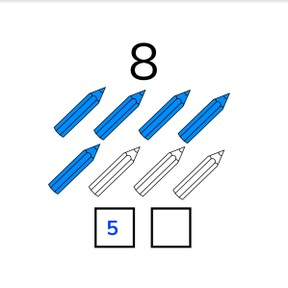Coloring decompositions to 10- recognizable objects

# Coloring decompositions to 10- recognizable objects

Coloring decompositions to 10- recognizable objects8,000 schools use Gynzy92,000 teachers use Gynzy1,600,000 students use Gynzy

## General

Students learn decomposition of numbers to 10 by coloring objects.

## Relevance

It is useful to be able to decompose numbers if you want to divide something into two groups. If you want to give six children lollypops, they might be in two groups of three. You then need to decompose your 6 lollipops into two groups, 3 and 3.

## Introduction

Students practice counting amounts to 10 by counting the different kinds of fruit.

## Development

You can decompose numbers in different ways. Using the apples, demonstrate that the number 6 can be decomposed into 1 and 5, but also 5 and 1. That is decomposing. If you already know one of the numbers, you only need to count on to discover the other number. Explain that this is composing a number. Then demonstrate decomposing the number 4 by coloring the snails step by step. Then show how you can decompose 5 by dividing the colored leaves on the two trees in different ways. Show all possible decompositions of 5. Explain that you can also switch numbers in a number bond. Practice coloring decompositions with students where the first number is given, and the second number must be figured out by the students.

Check that students understand decomposing with coloring to 10 by asking the following questions:
- What is decomposing numbers?
- How can you see what the decomposition is? (by looking at the colors in the picture)
- Can you give a few examples of decompositions of 10?

## Guided Practice

Students use a worksheet to practice coloring decompositions to 10. They take two colors and color the pictures. They also have to complete the number bond for each decomposition.

## Closing

Students color the decompositions and complete the number bond. They can come up with their own decompositions. Discuss that it is useful to be able to decompose numbers when you want to divide an amount into two groups.

## Teaching Tip

Students who are challenged by this goal can be supported by using manipulatives while determining the decompositions.

## Instruction materials

blocks

### The online teaching platform for interactive whiteboards and displays in schools

• Save time building lessons

• Manage the classroom more efficiently

• Increase student engagement